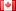# Revision history [back]

### Find specific linear combination in multivariare polynomial ring

Assume that I have given a sequence of polynomials $f_1,\dotsc,f_s$ in a multivariate polynomial ring (over $\mathbb{Z}$, if that matters) and want to decide whether a given polynomial $g$ can be written as $g = \lambda_1 f_1 + \dotsc + \lambda_s f_s$. Then in Sage I just let

I = Ideal([f_1,...,f_s])


and test with

g in I


If this returns True, how can I get Sage to display some possible $\lambda_1,\dotsc,\lambda_s$?

As for my specific problem, I have already tried it by hand, but this is hard: My polynomial ring has $15$ indeterminates and there are $s = 250$ polynomials.2 typoDSM5232 ●21 ●68 ●112

### Find specific linear combination in multivariare multivariate polynomial ring

Assume that I have given a sequence of polynomials $f_1,\dotsc,f_s$ in a multivariate polynomial ring (over $\mathbb{Z}$, if that matters) and want to decide whether a given polynomial $g$ can be written as $g = \lambda_1 f_1 + \dotsc + \lambda_s f_s$. Then in Sage I just let

I = Ideal([f_1,...,f_s])


and test with

g in I


If this returns True, how can I get Sage to display some possible $\lambda_1,\dotsc,\lambda_s$?

As for my specific problem, I have already tried it by hand, but this is hard: My polynomial ring has $15$ indeterminates and there are $s = 250$ polynomials.